Next: Methods of quality assessment Up: The FAMUSAMM algorithm Previous: Multiple Time Step Methods

## Combination of SAMM with a multiple-time-step method

Before starting with the detailed outline of our FAMUSAMM method we want to annotate on an important progress concerning its implementation as compared to that of SAMM: The first two SAMM versions [19, 18, 26] made use of only two hierarchy levels, which restricted their applicability to medium-sized systems comprising less than about 5,000 atoms. To gain substantial speed-ups also for larger systems, we decided for the design of FAMUSAMM to implement a fast SAMM method with an arbitrary number of hierarchy levels. That number is now determined by the number of atoms in a given simulation system and is chosen automatically in the start-up phase of a simulation .

a) Basic concepts
Our FAMUSAMM algorithm has been designed to further reduce the computational effort for the evaluation of Coulomb forces by exploiting regularities of these forces in space and time. The basis of its development has been the identification of the most time-consuming steps within the fast SAMM algorithm. Only upon such an analysis one may expect that a combination with a multiple-time-step method can render additional speed-ups: A detailed inquiry of fast SAMM has shown , that the essential time consuming steps are (i) the calculation of contributions to the local Taylor expansions (see Figure 2, steps 1 and 3) and (ii) the calculation of the electrostatic forces for the innermost distance class (see Figure 2, step 4). Therefore, we have focused our efforts on these two steps.

b) Multiple-time-step extrapolation of local Taylor expansions
First consider step (i) and remember, that the hierarchy of interactions, which is employed for the evaluation of the local Taylor expansions and is depicted in Figure 2, is defined by a hierarchy of distances. This hierarchy of distances is intimately related to the particular concept of distance classes underlying the multiple-time-step schemes as discussed above. Therefore, an attempt to combine these methods seems natural:

In multiple-time-step methods the total force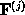acting on a selected atom originating from distance class j is extrapolated. Since we now want to use a multipole method, we have to identify a corresponding expression for this force. As we have seen in our outline of the SAMM algorithm, the total force originating from outer distance classes and acting on elements contained in a cluster or a structural unit is computed by means of a local Taylor expansion. Such a local Taylor expansion for an object on a given hierarchy level is -- except for the topmost level -- made up of two components: (a) a contribution inherited from the next higher hierarchy level (see Figure 2, step 2) comprising the electrostatic interactions with objects in all outer distance classes, and (b) contributions from objects within the same hierarchy level. From the latter one can calculate the desired total force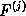and, therefore, we apply multiple-time-step extrapolations to these contributions. Here, instead of extrapolating the forces directly, we extrapolate the coefficients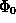,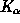and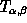of the local Taylor expansions, from which the forces derive.

c) Inner class force extrapolation
Because of the choice of 10Å for the interaction distance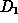rendering quite a few, typically about 400, interaction partners for a given partial charge within the innermost distance class (H=0) of SAMM, the second essential time consuming step (ii) of that method is the calculation of electrostatic forces by the Coulomb sum, eq. (1). In fact, this step is equivalent to the one carried out in conventional truncation methods using a cut-off distance. To reduce that effort by application of a multiple-time-step method, we split this SAMM distance class into two new distance classes j=0 and j=1 with the respective radii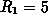Å and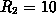Å. Within these new distance classes we extrapolate forces directly by a multiple-time-step scheme as described in the section before. Distance classes of similar size have been successfully used previously .

Due to the introduction of these new distance classes, the expensive computation of about 400 interactions per particle is now performed only at every second integration time step, whereas in distance class j=0 only a few interactions, about 50, have to be evaluated at every integration step. Hence, the overall computational effort for the innermost SAMM distance class is reduced nearly by a factor of two.

d) Interaction list updates
Generally, MD methods, which are based on distance classes, use interaction lists to eliminate the need for time consuming interaction partner searches at each integration time step . In FAMUSAMM that strategy is adopted as follows: For each object at a particular hierarchy level (atom, structural unit, cluster) an associated interaction list points to all objects, which belong to the same hierarchy level and meet the distance criterion described above (cf. Figure 2). Because distances between atoms, structural units and clusters vary during an MD simulation, the interaction lists have to be updated from time to time and are likely to change upon these updates. As a result, the forces generated by changing numbers of objects within a given distance class will change discontinuously. The reclustering procedure of SAMM mentioned above causes similar discontinuities. However, the occurrence of such discontinuities disables the application of multiple-time-step extrapolation procedures, since the latter are based on strict smoothness assumptions: Only if the interaction lists and cluster compositions are kept fixed for a sufficiently large [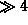, see subsection f)] number of integration time steps, multiple-time-step extrapolations may be applied. Test simulations have shown, that for our FAMUSAMM interaction lists update periods covering up to 128 integration steps can be used; reclustering periods may be even longer and are chosen as integer multiples of the former periods.

e) Verification of local Taylor expansion smoothness
As described above, an essential new feature of FAMUSAMM as compared to previous multiple-time-step schemes is the extrapolation of local Taylor expansion coefficients for outer distance classes instead of the extrapolation of forces acting on individual atoms. To verify the validity of this approach it remains to be checked, whether the smoothness conditions required for multiple-time-step extrapolations actually hold for the local Taylor expansion coefficients. Extended test simulations have demonstrated, that this is the case, indeed, and that local Taylor expansion coefficients exhibit decreasing amplitudes and curvatures with increasing hierarchy level H.

f) Initialization
A prerequisite for the application of the multiple-time-step extrapolation at a given time step in a simulation is the accessibility to two previously explicitly calculated forces or local Taylor expansion coefficients. As these are absent in the start-up phase of a simulation or, due to the associated discontinuities, cannot be used after an update of the interaction lists, special precautions have to be taken for these periods . To be specific, the extrapolation of forces or of local coefficients for distance class j requires their values at two previous macro integration steps which are separated by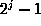basic integration steps (see Figure 3). To provide these values for the initialization periods FAMUSAMM performs exactly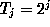conventional fast SAMM steps until all necessary forces (for j=1) or local coefficients (for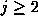) are explicitly calculated. Obviously, the initialization periods, after which multiple-time-step extrapolations can be applied, have different durations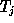for the various distance classes. For the computationally most expensive distance classes, j=1 and j=2, the initialization lasts only 2 and 4 integration steps, respectively. From these numbers it is clear that the efficiency loss due to the initialization phases is negligible, if periods of 64 or more steps are used for the update of the interaction lists.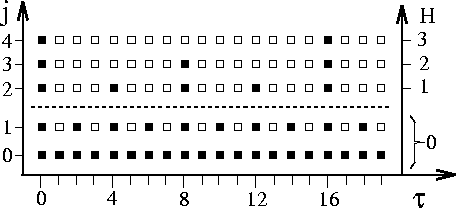Figure 4: Extrapolation scheme of FAMUSAMM;denotes the integration step, H the SAMM hierarchy level and j the multiple-time-step distance class; filled squares mark the integration steps at which forces originating from objects in distance class j are explicitly calculated, whereas empty squares indicate computationally inexpensive extrapolations. The dashed lines separate the regime of force extrapolation in distance class j=1 (H=0) from the regime of local Taylor expansion extrapolation in the outer distance classes(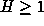); forces originating from partial charges in the innermost distance class j=0 are never extrapolated.

g) The FAMUSAMM algorithm
Figure 4 illustrates the multiple-time-step scheme of FAMUSAMM by which long range Coulomb interactions are evaluated. For a simulation period well after an initialization phase three hierarchy levels H and a sequence of 20 integration steps are shown. Filled squares indicate explicit fast SAMM force calculations, empty squares extrapolations. The dashed line separates the Coulomb sum interaction classes (j=0,1) from distance classes which interact by multipole expansions (). For class j=1 the extrapolation of forces is used, i.e., the respective forces are evaluated only at every second integration step and are extrapolated otherwise; whereas, the electrostatic interactions of partially charged atoms closer thanÅ (see Figure 3, j=0) are calculated at every integration step.

For distance classes, which correspond to hierarchy levels, the multiple-time-step scheme is applied to the local Taylor expansion coefficients. Here, the local Taylor expansion coefficients of lower hierarchy levels have to be recalculated more often than those of higher hierarchy levels, which, in turn, can be extrapolated more often. As suggested in a previous multiple-time-step method , explicit recalculation is performed every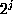-th integration step.

Appendix A summarizes the FAMUSAMM algorithm using a pseudo-code description. The algorithm has been implemented in the MD simulation program EGO_VIII  in a sequential and a parallelized version; the latter is suited for distributed memory parallel computers, e.g., IBM SP2, Cray T3D, Parsytec CC and workstation clusters under PVM or MPI. Details on the parallelization strategy will be given in a forthcoming publication .

As is apparent from the large number of empty squares depicted in Figure 4 FAMUSAMM replaces quite a few explicit force or local Taylor expansion calculations by extrapolations for the sole purpose of computational efficiency. As noted already in the introduction, in MD-simulations the inaccuracies associated with these FAMUSAMM approximations may effectively act as sources of uncontrolled algorithmic noise. It is the aim of the remaining sections of this paper to check, to what extent such numerical artifacts can affect the MD description of structural and dynamical properties of proteins.Next: Methods of quality assessment Up: The FAMUSAMM algorithm Previous: Multiple Time Step Methods

Helmut Grubmueller
Wed Apr 30 15:40:09 MET DST 1997